# Legacy DocumentImportant: The information in this document is obsolete and should not be used for new development.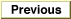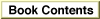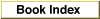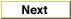Inside Macintosh: Imaging With QuickDraw /
Chapter 3 - QuickDraw Drawing / QuickDraw Drawing Reference
Routines / Creating and Managing Rectangles

### PtToAngle

To calculate an angle between a vertical line pointing straight up from the center of a rectangle and a line from the center to a given point, use the `PtToAngle` procedure.

```PROCEDURE PtToAngle (r:\xDDRect; pt: Point; VAR angle: Integer);
```
`r`
The rectangle to examine.
`pt`
The point to which an angle is to be calculated.
`angle`
The resulting angle.
##### DESCRIPTION
The `PtToAngle` procedure returns in the `angle` parameter the angle between a vertical line (pointing straight up from the center of the rectangle that you specify in the `r` parameter) and a line from the center of that rectangle to a point (which you specify in the `pt` parameter).

The result returned in the `angle` parameter is specified in degrees from 0 to 359, measured clockwise from 12 o'clock, with 90 at 3 o'clock, 180 at 6 o'clock, and 270 at 9 o'clock. Other angles are measured relative to the rectangle. If the line to the given point goes through the upper-right corner of the rectangle, the angle returned is 45, even if the rectangle isn't square; if it goes through the lower-right corner, the angle is 135, and so on, as shown in Figure 3-18.

Figure 3-18 Forty-five-degree angles as returned by the `PtToAngle` procedure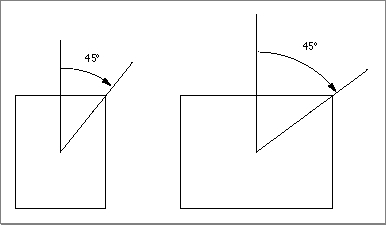The angle returned might be used as input to one of the procedures that manipulate arcs and wedges, as described in "Drawing Arcs and Wedges" beginning on page 3-67.